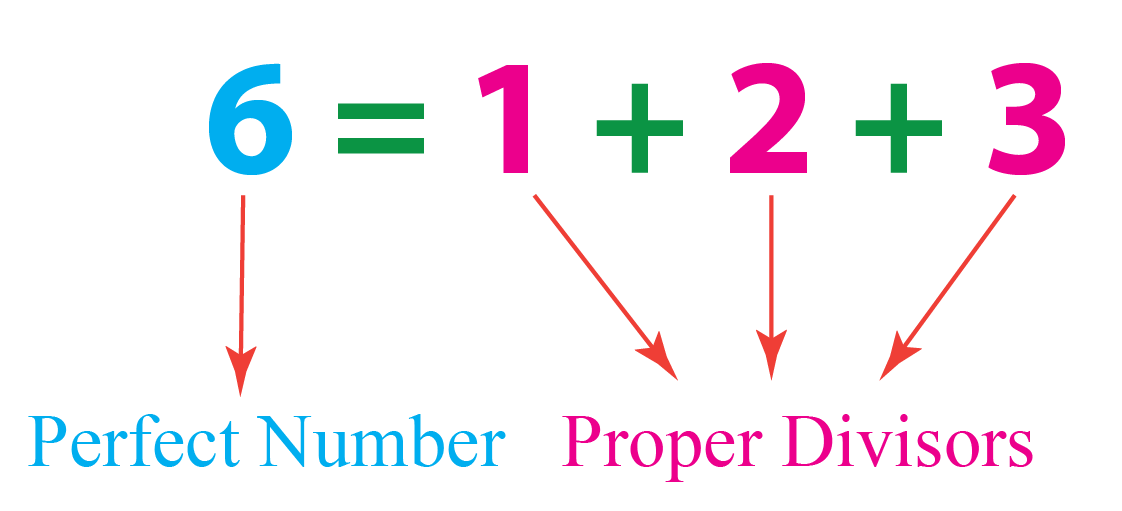# Perfect Numbers

Perfect Numbers
Go back to  'Numbers'

 1 History of Perfect Numbers 2 What are Perfect Numbers? 3 Perfect Numbers List in a Table 4 How to Find a Perfect Number? 5 Tips and Tricks 6 Solved Examples of Perfect Numbers 7 Important Notes on Perfect Numbers 8 Practice Questions on Perfect Numbers 9 Frequently Asked Questions (FAQs)

We at Cuemath believe that Math is a life skill. Our Math Experts focus on the “Why” behind the “What.” Students can explore from a huge range of interactive worksheets, visuals, simulations, practice tests, and more to understand a concept in depth.

Book a FREE trial class today! and experience Cuemath's LIVE Online Class with your child.

## History of Perfect Numbers

There is not much information regarding the discovery of perfect numbers.

It is said that perhaps the Egyptians may have discovered them.

Despite knowing the existence of perfect numbers, it was only the Greeks who were eager to study more about these numbers.

Perfect numbers were studied by Pythagoras and his followers for its mystical properties.

The smallest perfect number found was 6

It is still unknown whether there are infinite perfect numbers.

## What are Perfect Numbers?

Perfect numbers are the positive integers which are equal to the sum of its factors except the number itself.

In other words, perfect numbers are the positive integers which are the sum of its proper divisors.

The smallest perfect number is 6, which is the sum of its proper divisors: 1, 2 and 3Do you know that when the sum of all divisors of a number is equal to twice the number, the number has a separate name?

Such numbers are called complete numbers.

In fact, all perfect numbers are also complete numbers.

## Perfect Numbers List in a Table

The four perfect numbers 6, 28, 496 and 8128 are known to us since ancient times.

Perfect Number Sum of Divisors
6 1 + 2 + 3
28 1 + 2 + 4 + 7 + 14
496 1 + 2 + 4 + 8 + 16 + 31 + 62 + 124 + 248
8128 1 + 2 + 4 + 8 + 16 + 32 + 64 + 127 + 254 + 508 + 1016 + 2032 + 4064

Experiment with the simulation below to check whether the number shown is perfect or not.

CLUEless in Math? Check out how CUEMATH Teachers will explain Perfect Numbers to your kid using interactive simulations & worksheets so they never have to memorise anything in Math again!

Explore Cuemath Live, Interactive & Personalised Online Classes to make your kid a Math Expert. Book a FREE trial class today!

## How to Find a Perfect Number?

There are many propositions to find perfect numbers.

Some of them are given below.

### Proposition 1

If as many numbers as those beginning from unit be set out continuously in double proportion until the sum of all becomes a prime, then the product of the sum and the last number makes a perfect number.

Double Proportion means that each number in a sequence is double the preceding number.

For example,

$$1+2+4=7$$ which is a prime number.

According to Proposition 1,

\begin{align}(\text{sum}) \!\!\times \! (\text{last number})\!&=\!(\text{perfect number})\\7\!\times \!4=28\end{align}

And yes! 28 is a perfect number.

### Proposition 2

If N is a Mersenne prime, then \begin{align}\frac{N(N+1)}{2}\end{align} is a perfect number.

Mersenne prime is a prime that is one less than the power of 2

For example,

Let's take $$N$$ as 31 which is 1 less than $$2^4$$

Then,

\begin{align}\frac{N(N+1)}{2}=\frac{31(31+1)}{2}=496\end{align}

And 496 is a perfect number.Tips and Tricks
1. The $$k^{\text{th}}$$ perfect number has $$k$$ digits.
2. All perfect numbers are even. It is still unknown whether odd perfect numbers exist or not.
3. All perfect numbers end in 6 and 8 alternatively.
4. The sum of all divisors of a perfect number gives the result as twice the perfect number.

Help your child score higher with Cuemath’s proprietary FREE Diagnostic Test. Get access to detailed reports, customized learning plans, and a FREE counseling session. Attempt the test now.

## Solved Examples

Look at the examples of perfect numbers below.

 Example 1

Is 28 a perfect number?

Solution:

The proper factors of 28 are 1, 2, 4, 7 and 14

The sum of proper factors is 28

According to the definition of perfect numbers, 28 is a perfect number.

 $$\therefore$$ Yes, 28 is a perfect number.
 Example 2

According to the Euclid's proposition, if $$2^p -1$$ is a prime number, then $$2^{p-1}(2^{p}-1)$$ is a perfect number.

Can you make a list of 8 perfect numbers using this proposition?

Solution:

The first 8 Mersenne Primes are 2, 3, 5, 7, 13, 17, 19 and 31

Prime, $$p$$ $$2^{p}-1$$ Perfect Number, $$2^{p-1}(2^{p}-1)$$
2 3 6
3 7 28
5 31 496
7 127 8128
13 8191 33550336
17 131071 8589869056
19 524287 137438691328
31 2147483647 2305843008139952128
 $$\therefore$$  Above mentioned are the first 8 perfect numbers.

More Important Topics
Numbers
Algebra
Geometry
Measurement
Money
Data
Trigonometry
Calculus